﻿ 考虑接触的斜裂纹动态应力强度因子分析
«上一篇文章快速检索 高级检索

 哈尔滨工程大学学报2019, Vol. 40Issue (8): 1399-1405  DOI: 10.11990/jheu.2018080820

### 引用本文PENG Ying, YANG Ping, JIANG Wei. Analysis of dynamic stress intensity factors for inclined cracks considering contact interaction[J]. Journal of Harbin Engineering University, 2019, 40(8), 1399-1405. DOI: 10.11990/jheu.201808082.### 文章历史

1. 武汉理工大学 交通学院, 湖北 武汉 430063;
2. 武汉理工大学 高性能船舶技术教育部重点实验室, 湖北 武汉 430063

Analysis of dynamic stress intensity factors for inclined cracks considering contact interaction
PENG Ying 1, YANG Ping 1,2, JIANG Wei 1
1. School of Transportation, Wuhan University of Technology, Wuhan 430063, China;
2. Key Laboratory of High Performance Ship Technology(Wuhan University of Technology), Ministry of Education, Wuhan 430063, China
Abstract: A series of models was analyzed by using the finite element method to investigate the dynamic stress intensity factors (DSIFs) at the crack tip of a centrally cracked finite plate under tension impact loading. The stress singularity at the crack tip was simulated by quarter-point singular elements. DSIFs were obtained through the interaction integral method. The influences of crack contact, impact load style, crack length, crack angle, and crack location were discussed. The time history curves of contact stresses were also presented. Numerical results showed that the negative DSIFs for mode Ⅰ can be eliminated if the contact between crack faces was considered. The influence of contact stiffness on DSIFs was more pronounced than that of the friction coefficient, and the influence of load style was significant even if the total impulse remained constant. The effect of crack parameters, such as crack length, on the DSIFs for mode Ⅰ was more significant than that on the DSIFs for mode Ⅱ.
Keywords: inclined crack    tension impact loading    dynamic stress intensity factors    interaction integral    crack contact    load style    crack parameters    contact stresses

1 基本理论

 $I=\int_{\mathit{\Gamma}}\left[\boldsymbol{W}^{(1, 2)} \delta_{1 j}-\sigma_{i j}^{(1)} \frac{\partial\left(u_{i}^{(2)}\right)}{\partial x_{1}}-\sigma_{i j}^{(2)} \frac{\partial\left(u_{i}^{(1)}\right)}{\partial x_{1}}\right] n_{j} \mathrm{d} \mathit{\Gamma}$ (1)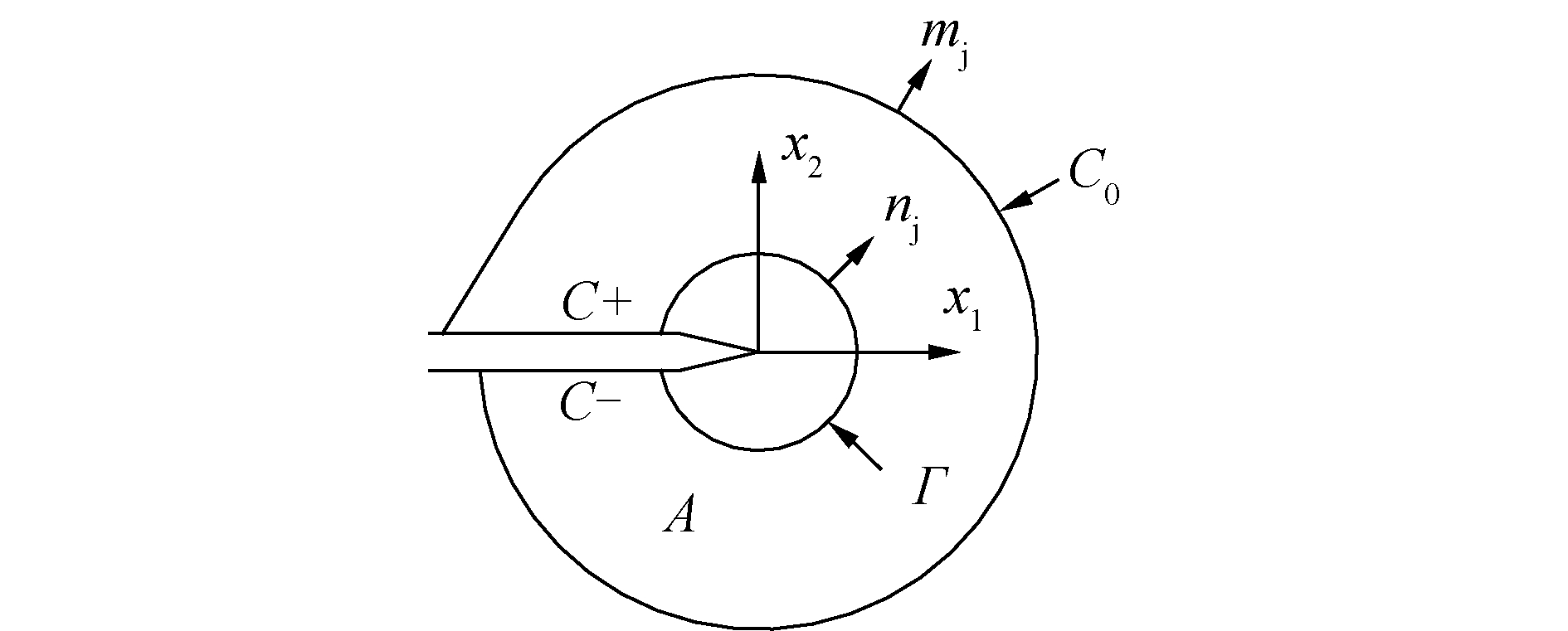Download: 图 1 相互作用积分示意 Fig. 1 The diagram of interaction integral

 $I=\frac{2}{E^{*}}\left(K_{\mathrm{I}}^{(1)} K_{\mathrm{I}}^{(2)}+K_{\mathrm{II}}^{(1)} K_{\mathrm{II}}^{(2)}\right)$ (2)

 ${E^*} = \left\{ {\begin{array}{*{20}{c}} {E, ~~~~平面应力}\\ {\frac{E}{{1 - {v^2}}}, ~~~~平面应变} \end{array}} \right.$ (3)

K(2)=1，K(2)=0和K(2)=0，K(2)=1分别代入式(2)中，可求得Ⅰ型和Ⅱ型应力强度因子：

 $K_{\mathrm{I}}=\frac{E^{*}}{2} I^{(1)}, K_{\mathrm{II}}=\frac{E^{*}}{2} I^{(2)}$ (4)
2 计算模型

 $K_{{\mathrm{I}d}}^{n}=K_{{\mathrm{I}d}}(t) / K_{{\mathrm{I}s}}, K_{{\mathrm{II}d}}^{n}=K_{{\mathrm{II}d}}(t) / K_{I s}$ (5)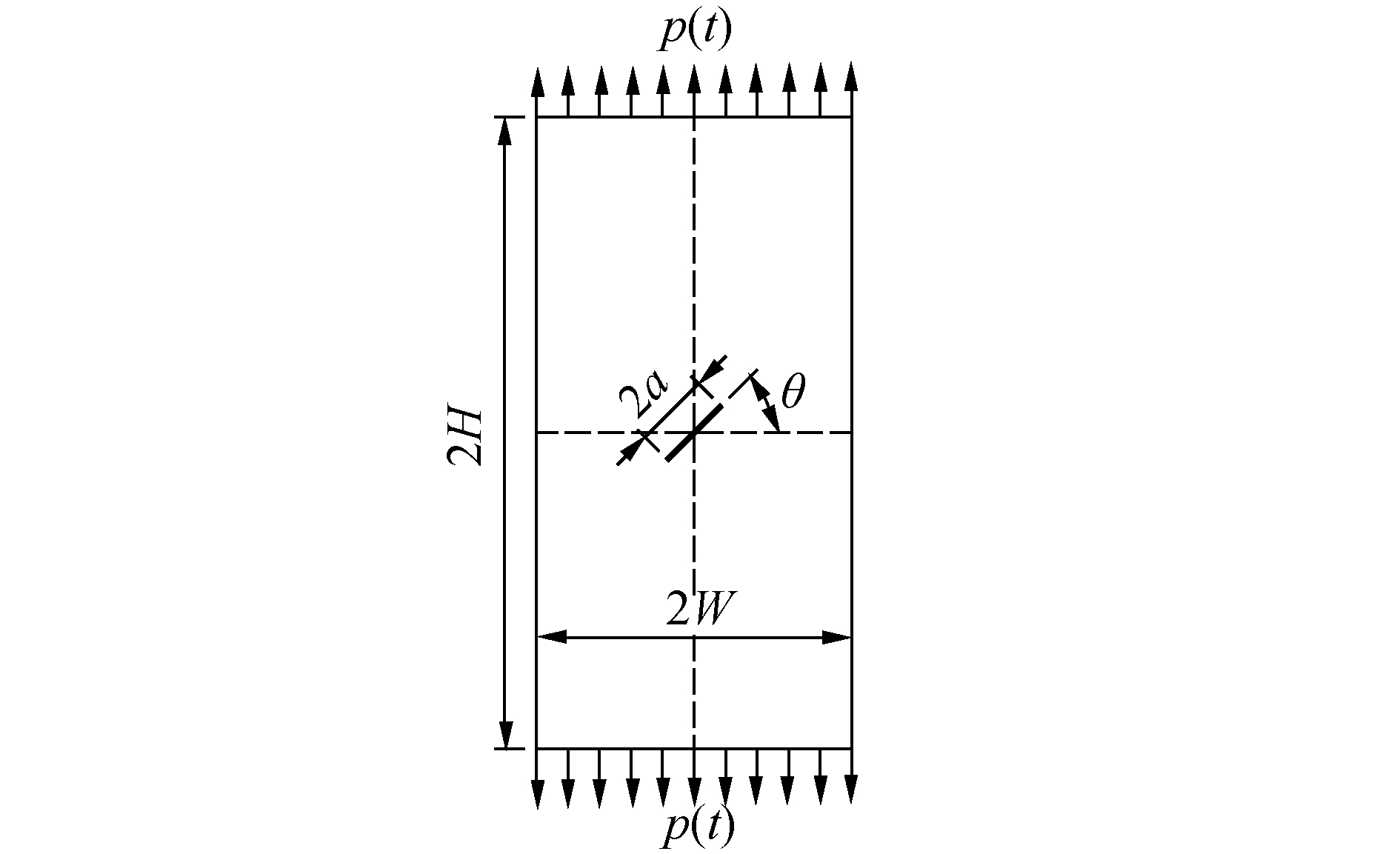Download: 图 2 中心穿透斜裂纹板几何模型 Fig. 2 Geometric model of finite plate with a central through inclined crack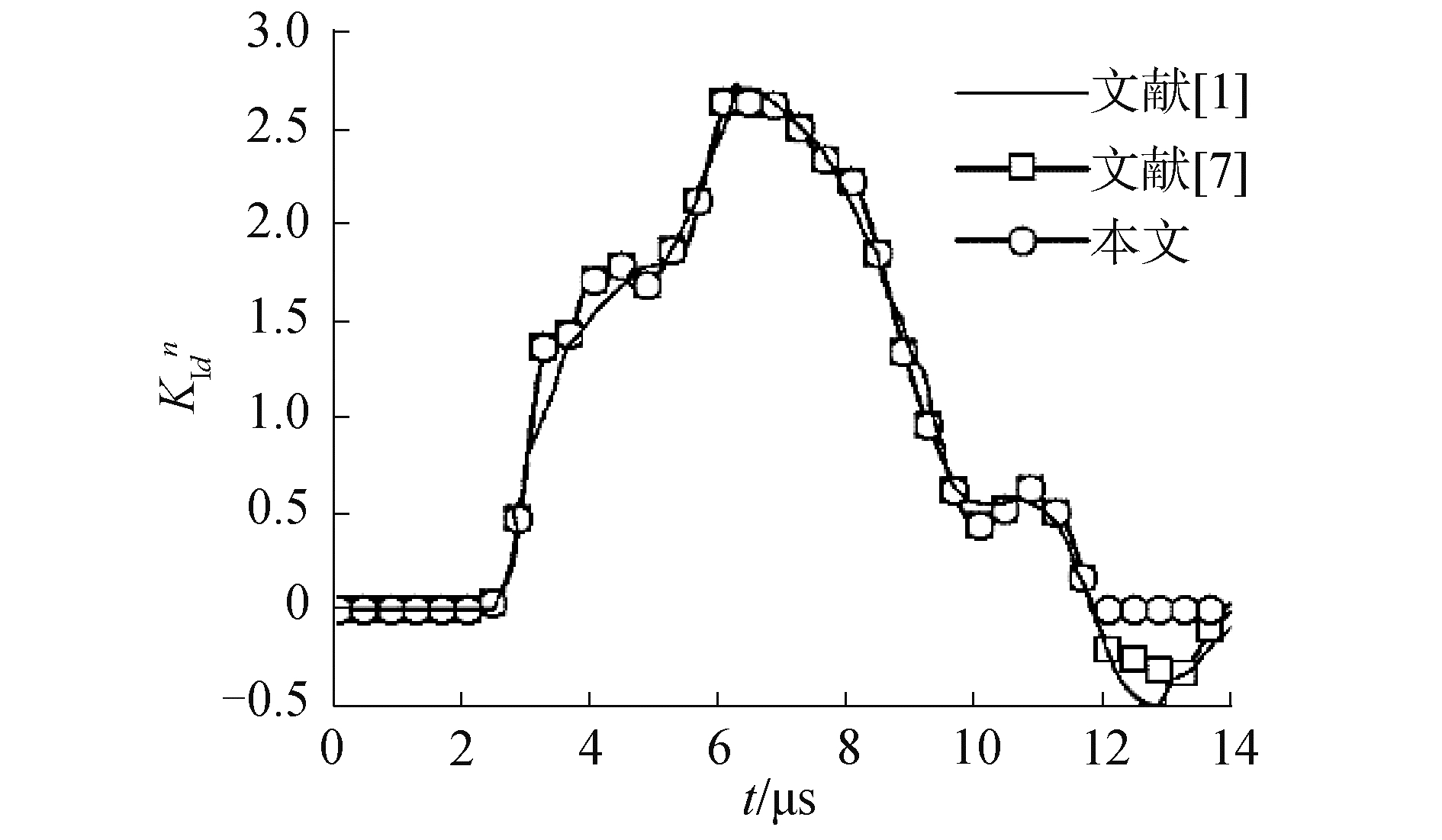Download: 图 3 本文计算结果与Chen等结果的对比 Fig. 3 Comparison between the present result and the reference solutions by Chen
3 DSIFs的影响参数分析

3.1 接触刚度kn对DSIFs的影响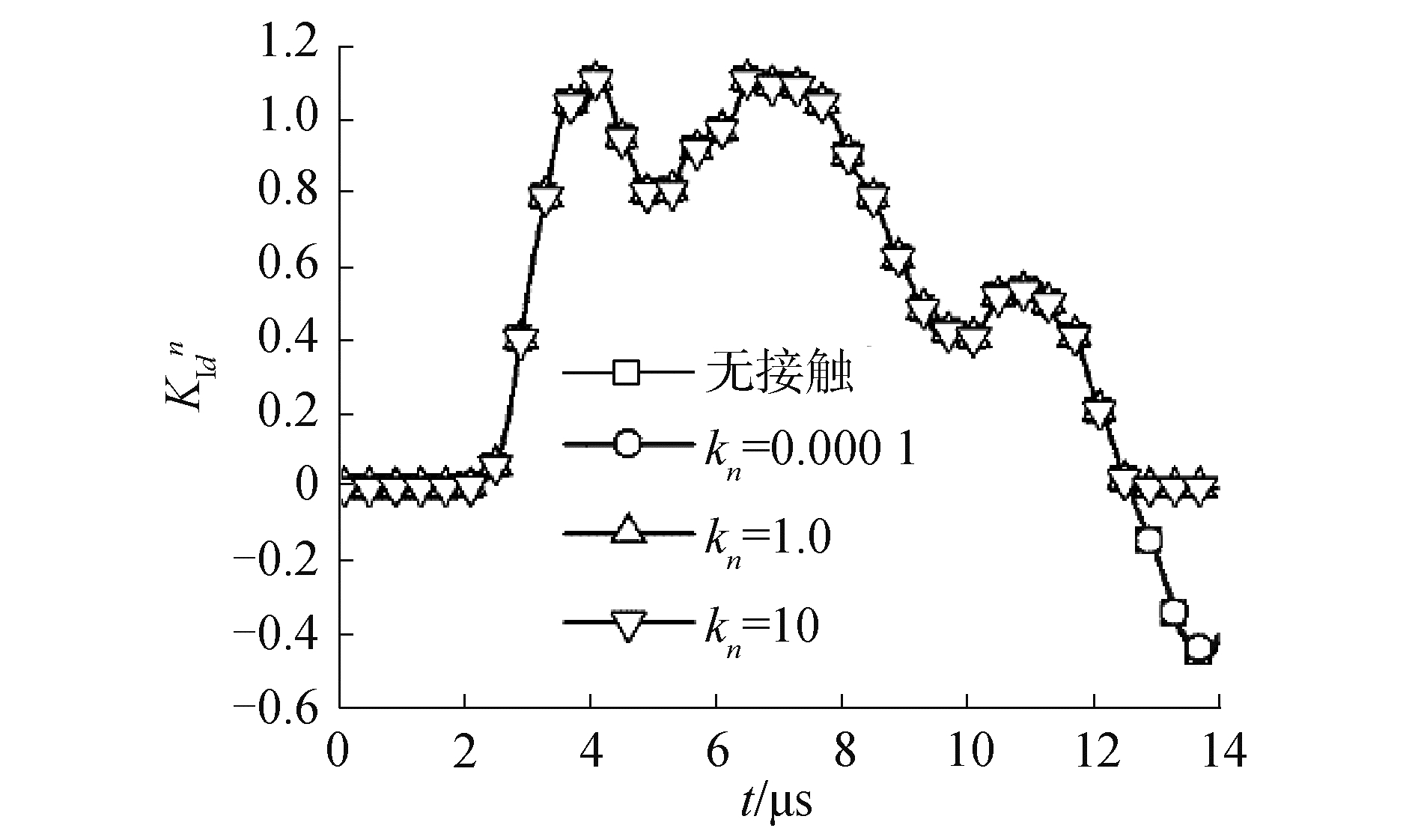Download: 图 4 不同接触刚度下的无量纲KⅠ(t)曲线 Fig. 4 Normalized KⅠ(t) for different contact stiffness
3.2 摩擦系数μ对DSIFs的影响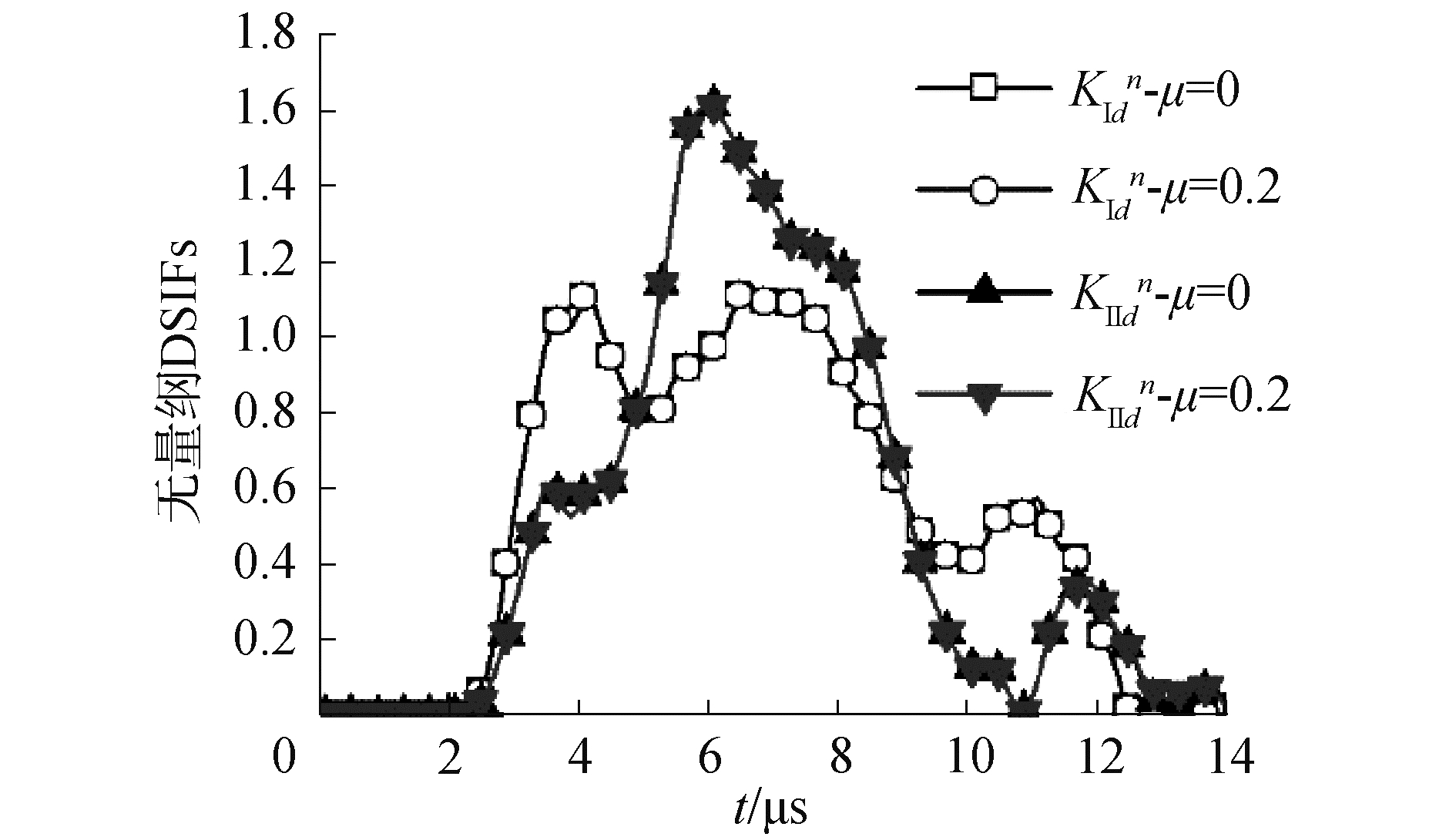Download: 图 5 不同摩擦系数下的无量纲KⅠ(t)、KⅡ(t)曲线 Fig. 5 Normalized KⅠ(t) and KⅡ(t) for different friction coefficients
3.3 载荷形式对DSIFs的影响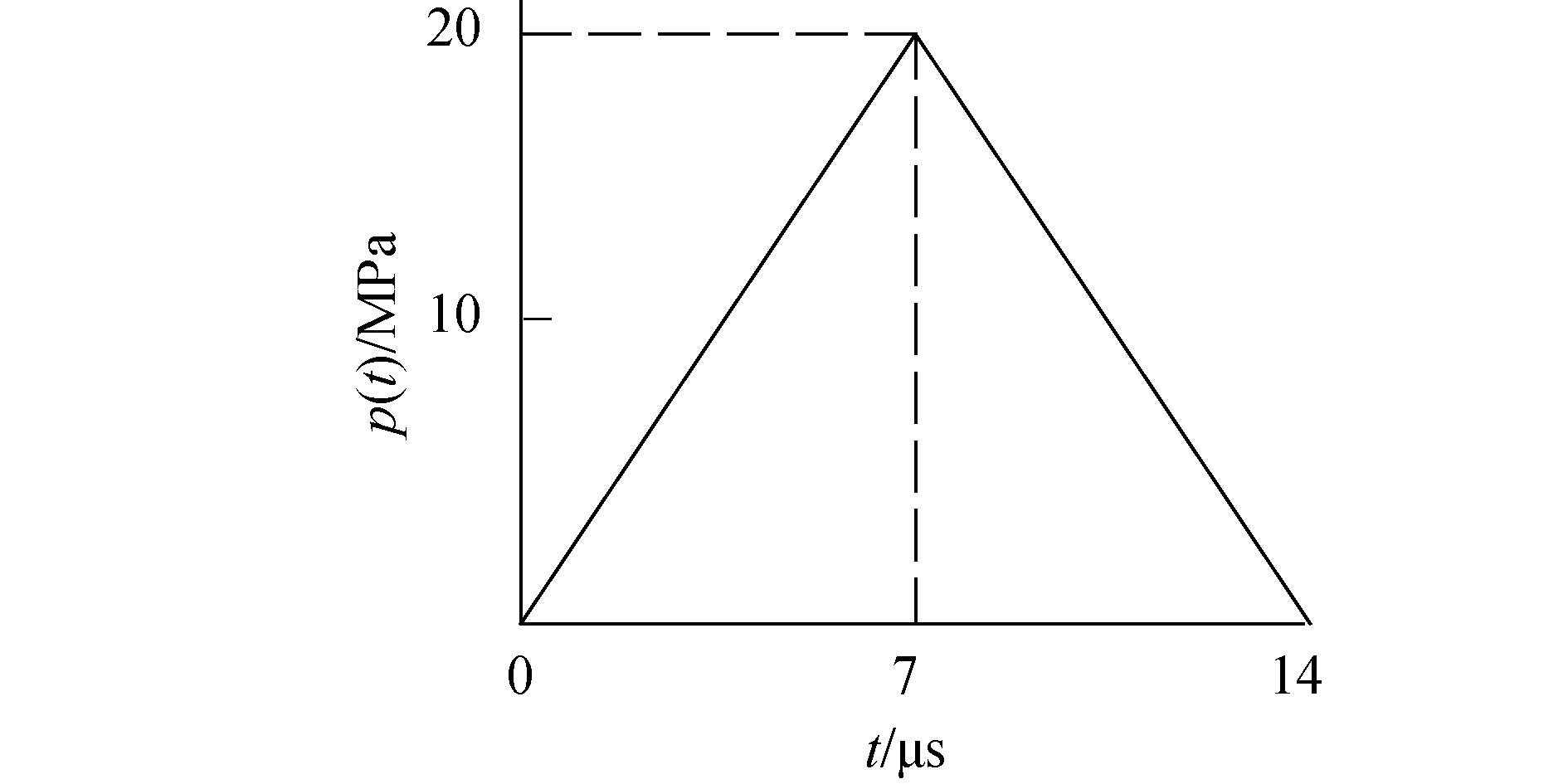Download: 图 6 三角形冲击载荷 Fig. 6 Triangular impact loading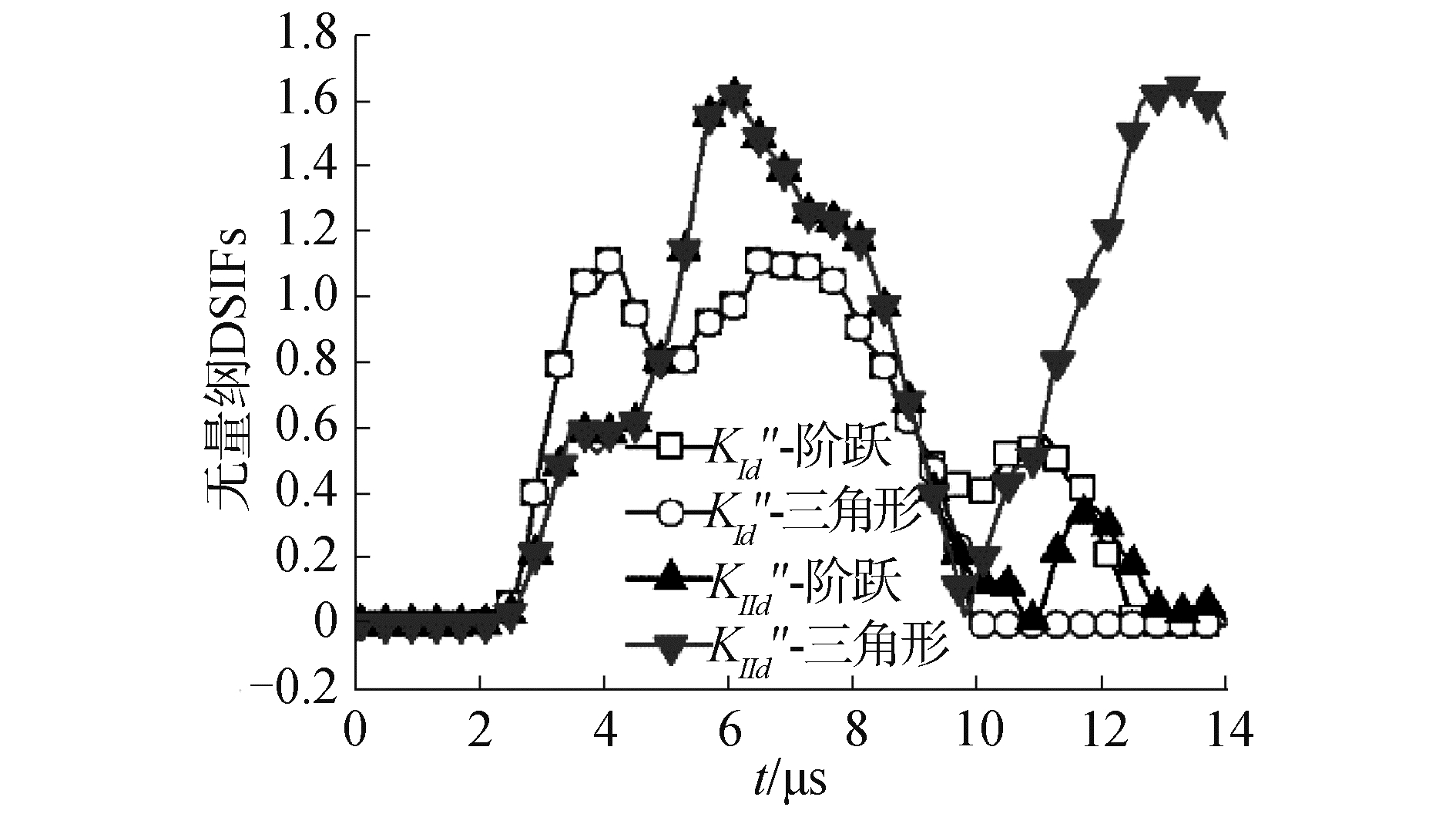Download: 图 7 不同载荷形式下的无量纲DSIFs曲线 Fig. 7 Normalized KⅠ(t) and KⅡ(t) for different load styles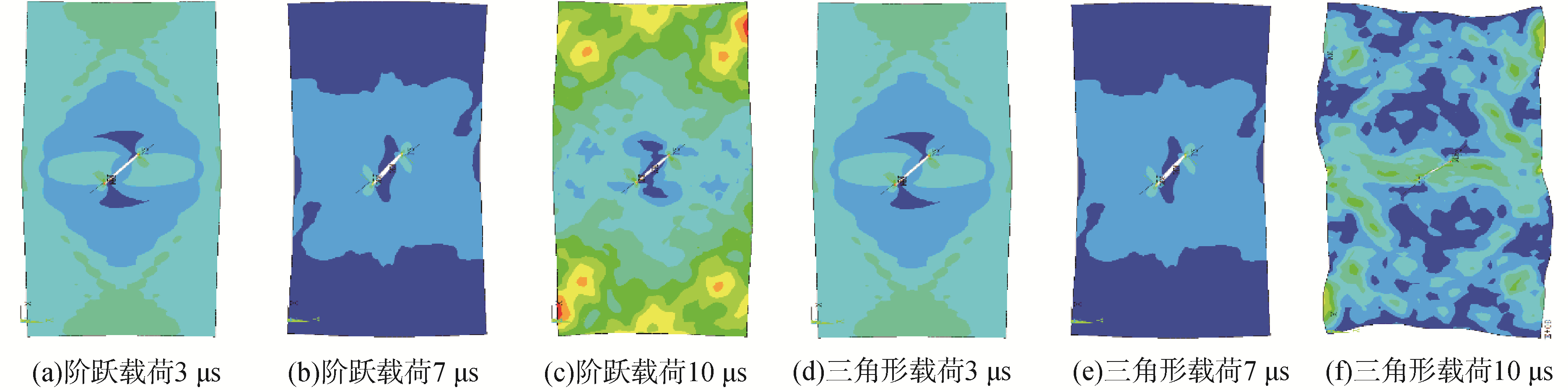Download: 图 8 不同载荷形式下不同时刻板的von-Mises应力云图 Fig. 8 Von-Mises stress distributions at different times for different load styles
3.4 裂纹长度对DSIFs的影响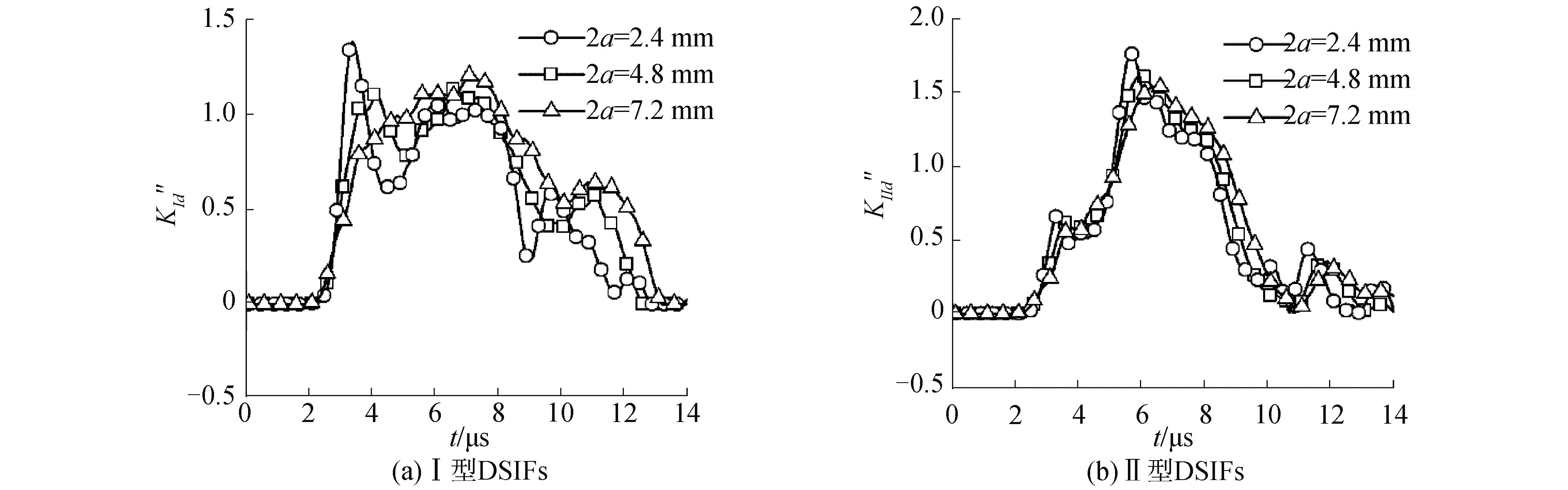Download: 图 9 不同裂纹长度下的无量纲DSIFs曲线 Fig. 9 Normalized DSIFs for different crack lengthDownload: 图 10 不同裂纹长度下不同时刻板的von-Mises应力云图 Fig. 10 Von-Mises stress distributions at different times for different crack length
3.5 裂纹角度对DSIFs的影响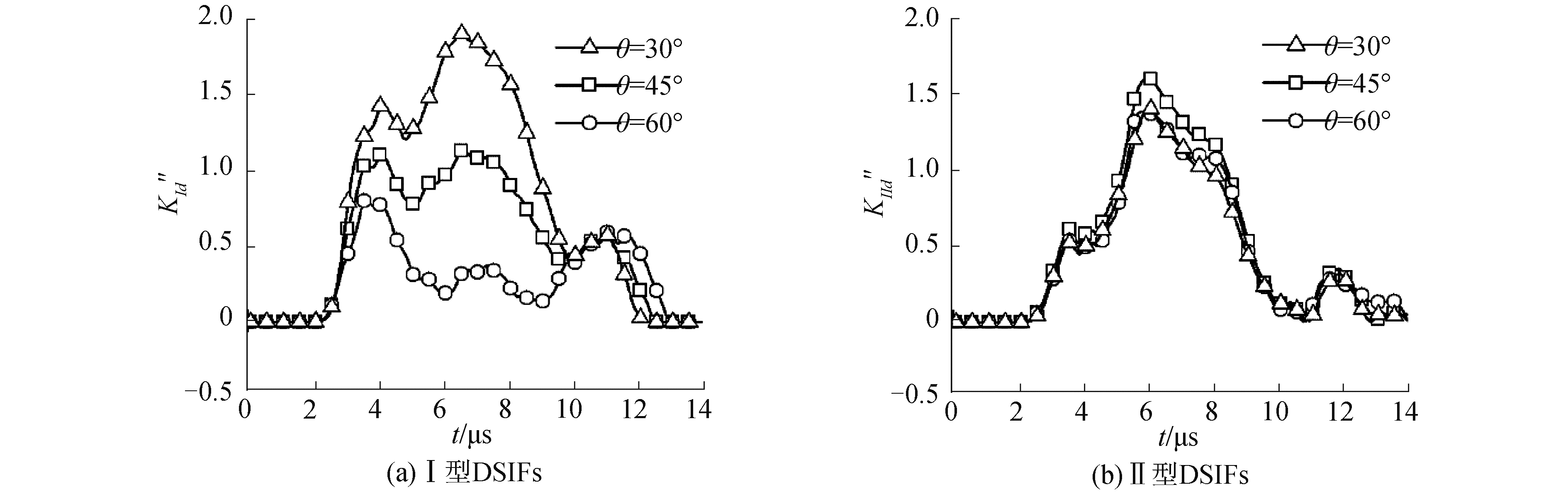Download: 图 11 不同裂纹角度下的无量纲DSIFs曲线 Fig. 11 Normalized DSIFs for different crack anglesDownload: 图 12 不同裂纹角度下不同时刻板的von-Mises应力云图 Fig. 12 Von-Mises stress distributions at different times for different crack angles
3.6 裂纹位置对DSIFs的影响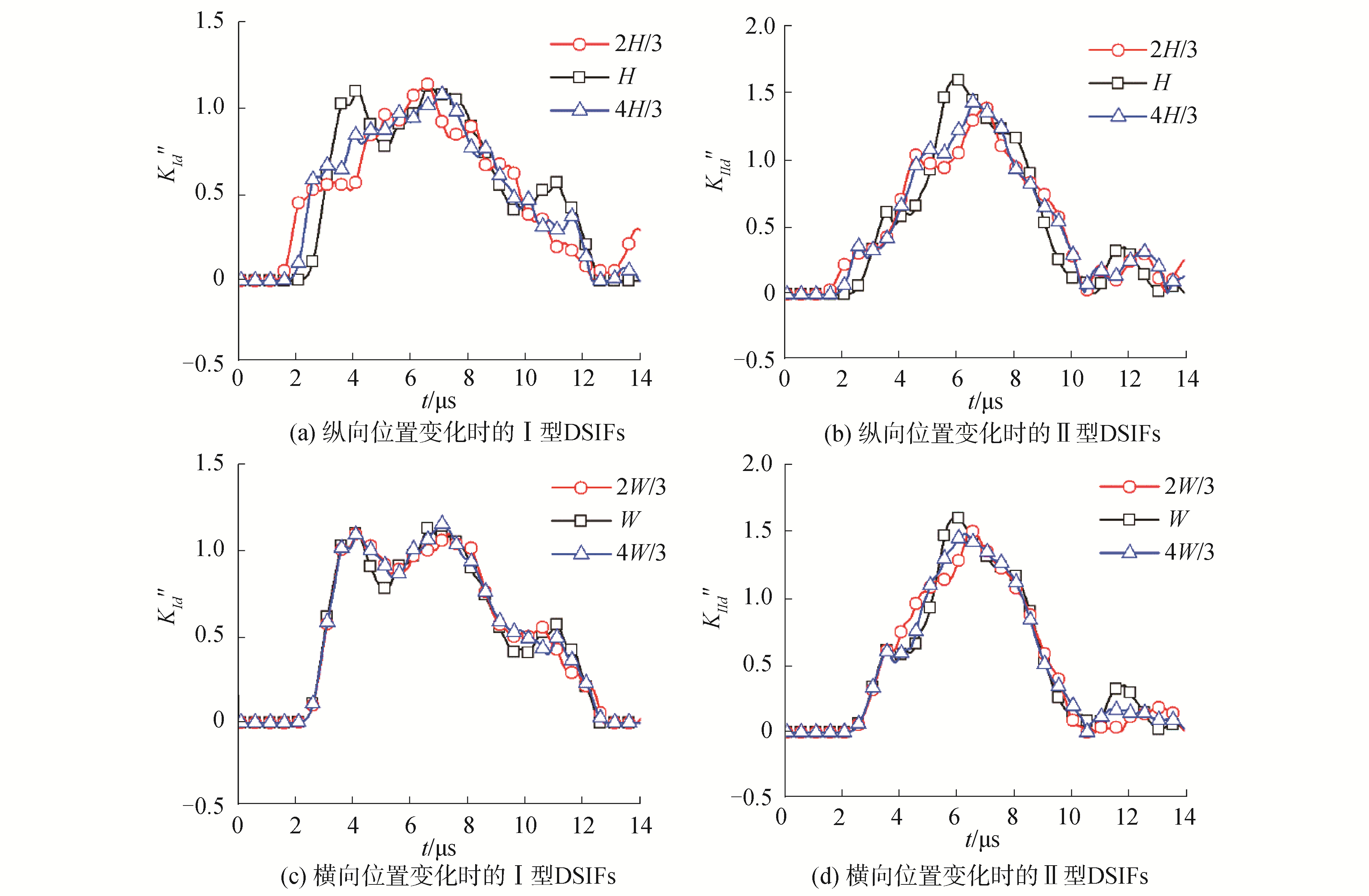Download: 图 13 不同裂纹位置下的无量纲DSIFs曲线 Fig. 13 Normalized DSIFs for different crack locationsDownload: 图 14 纵向位置变化时不同时刻板的von-Mises应力云图 Fig. 14 Von-Mises stress distributions at different times for different crack longitudinal location
4 裂纹面接触应力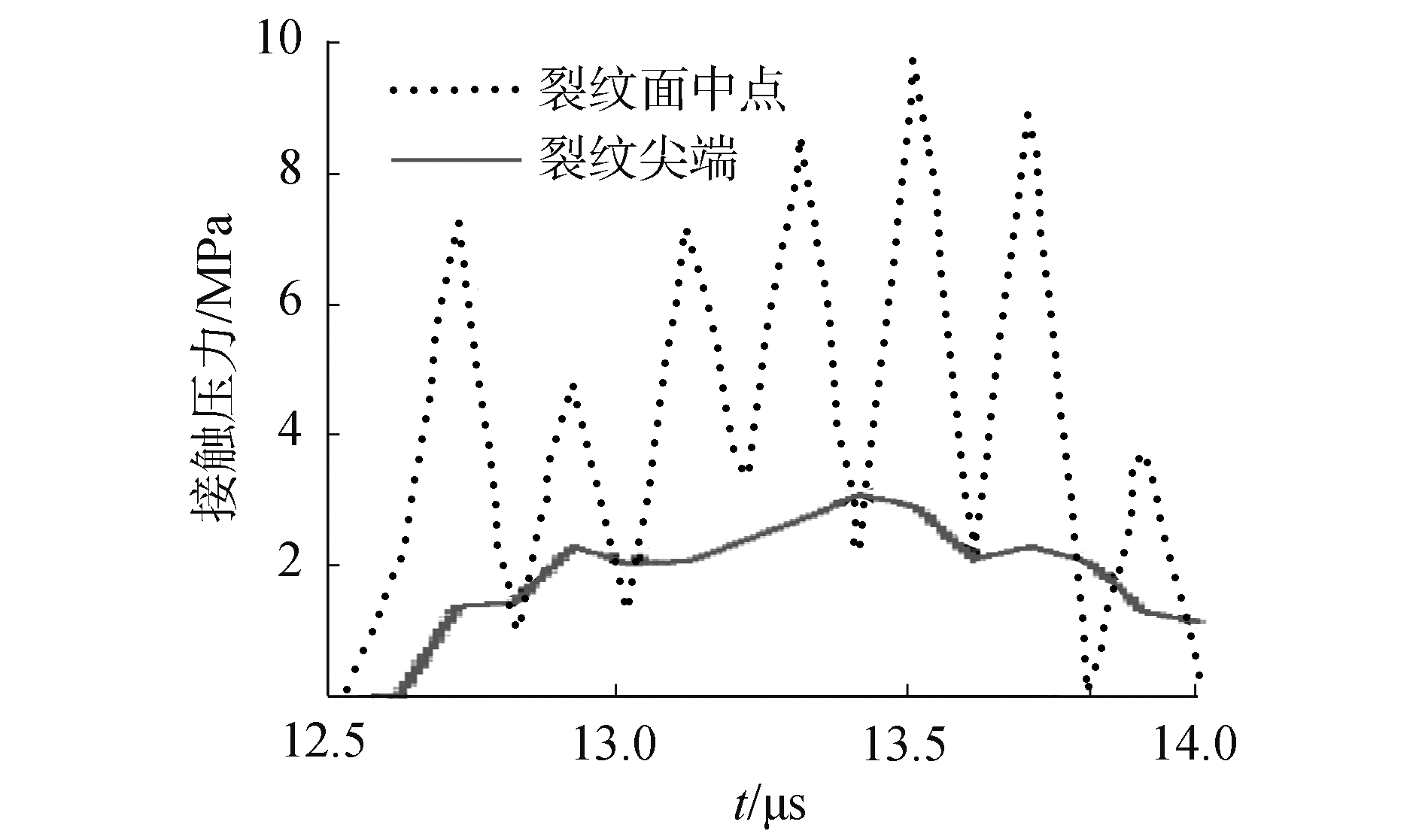Download: 图 15 裂纹面上随时间变化的接触压力 Fig. 15 Dynamic response curves of contact pressure on the crack faces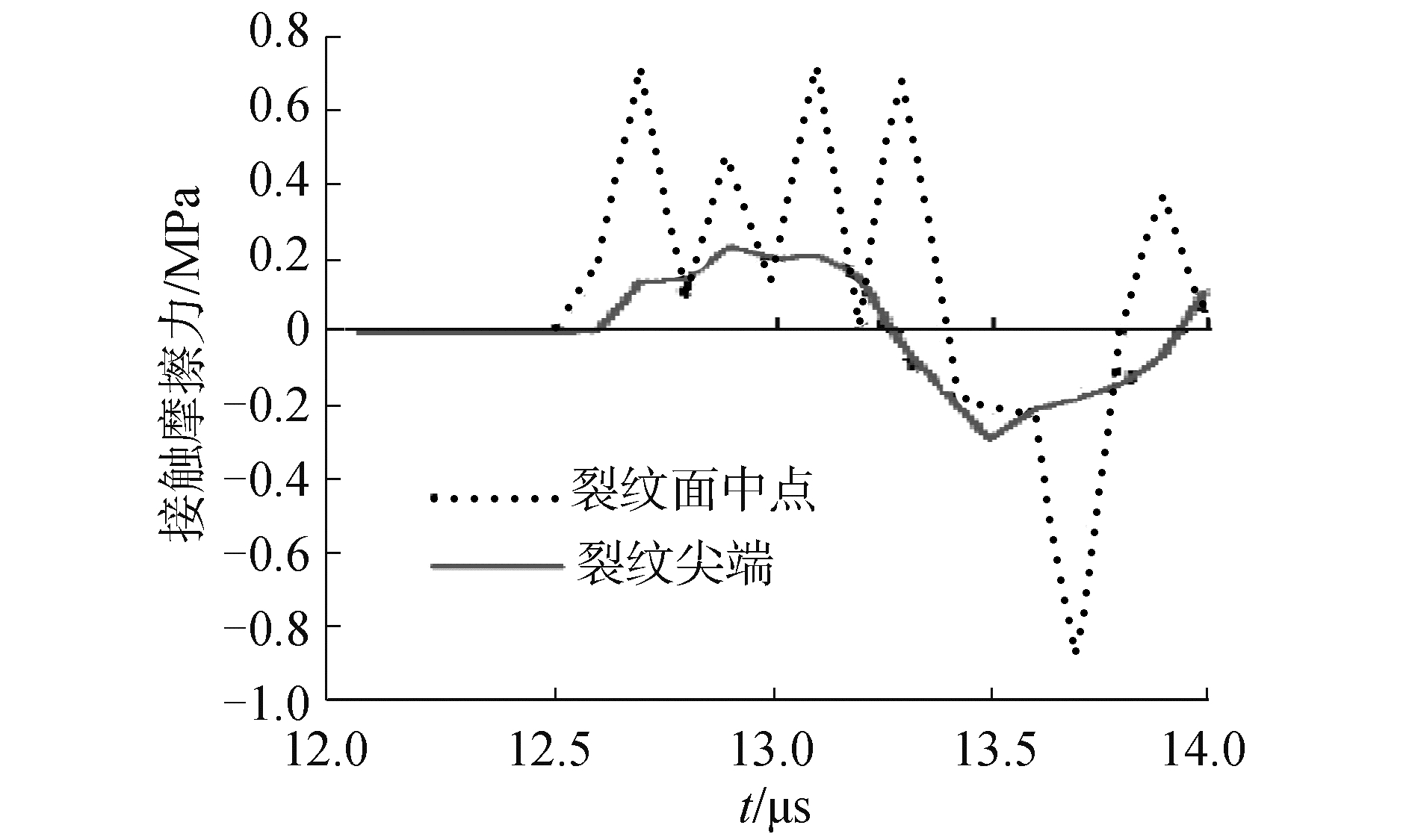Download: 图 16 裂纹面上随时间变化的接触摩擦力 Fig. 16 Dynamic response curves of contact friction stress on the crack faces

5 结论

1) 考虑裂纹面的接触作用，可以避免裂纹面发生相互穿透或重叠，从而消除了负Ⅰ型动态应力强度因子。

2) 选择合适的接触刚度对计算的收敛性和准确性十分重要，而摩擦系数对动态应力强度因子的影响在本算例中并不十分明显。载荷形式在总冲量不变的情况下对动态应力强度因子的影响也非常大，而裂纹长度等裂纹参数对Ⅰ型动态应力强度因子的影响比Ⅱ型显著。

3) 当裂纹面发生闭合接触时，接触压力迅速达到最大，相应的接触摩擦力也达到最大，这是符合实际情况的。

  CHEN Y M. Numerical computation of dynamic stress intensity factors by a Lagrangian finite-difference method (the HEMP code)[J]. Engineering fracture mechanics, 1975, 7(4): 653-660. DOI:10.1016/0013-7944(75)90021-1 (0)  LIN X, BALLMANN J. Re-consideration of Chen's problem by finite difference method[J]. Engineering fracture mechanics, 1993, 44(5): 735-739. DOI:10.1016/0013-7944(93)90202-4 (0)  SONG S H, PAULINO G H. Dynamic stress intensity factors for homogeneous and smoothly heterogeneous materials using the interaction integral method[J]. International journal of solids and structures, 2006, 43(16): 4830-4866. DOI:10.1016/j.ijsolstr.2005.06.102 (0)  SARIBAY M, NIED H F. Dynamic stress intensity factors for suddenly loaded structures using enriched finite element[J]. Theoretical and applied fracture mechanics, 2014, 70: 59-67. DOI:10.1016/j.tafmec.2014.02.003 (0)  IDESMAN A, BHUIYAN A, FOLEY J R. Accurate finite element simulation of stresses for stationary dynamic cracks under impact loading[J]. Finite elements in analysis and design, 2017, 126: 26-38. DOI:10.1016/j.finel.2016.12.004 (0)  解德, 钱勤, 李长安. 断裂力学中的数值计算方法及工程应用[M]. 北京: 科学出版社, 2009: 134-139. (0)  姜伟, 杨平, 董琴. 平板穿透裂纹尖端动态应力强度因子研究[J]. 武汉理工大学学报(交通科学与工程版), 2016, 40(5): 820-825. JIANG Wei, YANG Ping, DONG Qin. Research on dynamic stress intensity factors for through-cracked plates[J]. Journal of Wuhan University of Technology (transportation science & engineering), 2016, 40(5): 820-825. DOI:10.3963/j.issn.2095-3844.2016.05.012 (0)  王立清, 盖秉政. 有限板中圆孔边单边斜裂纹动态应力强度因子的数值计算[J]. 工程力学, 2009, 26(3): 9-14. WANG Liqing, GAI Bingzheng. Numerical calculation of dynamic stress intensity factors for a single hole-edge slant crack in a finite plate[J]. Engineering mechanics, 2009, 26(3): 9-14. (0)  王立清, 盖秉政. 裂纹面接触摩擦对双-边裂纹板动态应力强度因子的影响[J]. 工程力学, 2009, 26(7): 7-11. WANG Liqing, GAI Bingzheng. Effect of crack face contact and friction on dynamic stress intensity factors for a double-edge cracked plate[J]. Engineering mechanics, 2009, 26(7): 7-11. (0)  MOËS N, DOLBOW J, BELYTSCHKO T. A finite element method for crack growth without remeshing[J]. International journal for numerical methods in engineering, 1999, 46(1): 131-150. DOI:10.1002/(ISSN)1097-0207 (0)